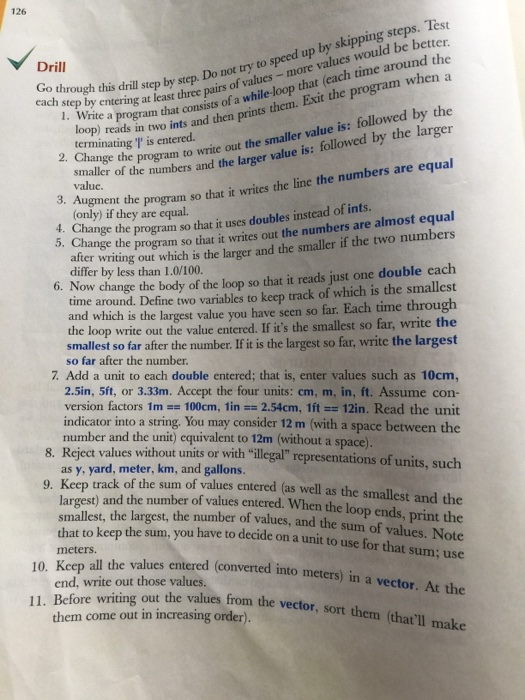# Answered! Question: Go through this drill step by step. Do not try to speed up by skipping steps. Test each step by e……C++

Go through this drill step by step. Do not try to speed up by skipping steps. Test each step by entering at least three pairs of values – more values would be better. Write a program that consists of a while-loop that (each time around the loop) reads in two ints and then prints them. Exit the program when a terminating is entered. Change the program to write out the smaller value is:followed by the smaller of the numbers and the larger value is:followed by the larger value. Augment the program so that it writes the line the numbers are equal (only) if they are equal. Change the program so that it uses doubles instead of ints. Change the program so that it writes out the numbers are almost equal after writing out which is the larger and the smaller if the two numbers differ by less than 1.0/100. Now change the body of the loop so that it reads just one double each time around. Define two variables to keep track of which is the smallest and which is the largest value you have seen so far. Each time through the loop write out the value entered. If it’s the smallest so far, write the smallest so far after the number. If it is the largest so far, write the largest so far after the number. Add a unit to each double entered:that is, enter values such as 10cm, 2.5in, 5ft, or 3.33m. Accept the four units:cm, m, in, ft. Assume conversion factors 1m == 100cm, 1in == 2.54cm, 1ft == 12in. Read the unit indicator into a string. You may consider 12 m (with a space between the number and the unit) equivalent to 12m (without a space) Reject values without units or with “illegal” representations of units such as y, yard, meter, km, and gallons. Keep track of the sum of values entered (as well as the largest) and the number of values entered. When the loop ends, print the smallest, the largest, the number of values, and the sum of values. Keep all the values entered (convened into meters) in a vector. At the end, write out those values. Before writing out the values from die vector, sort them (that’ll make them come out in increasing order).

Don't use plagiarized sources. Get Your Custom Essay on
Answered! Question: Go through this drill step by step. Do not try to speed up by skipping steps. Test each step by e……
GET AN ESSAY WRITTEN FOR YOU FROM AS LOW AS \$13/PAGE

ans 1: #include<iostream>

using namespace std;
int main()
{
int a,b;
cin>>a>>b;
while(a!=’|’&&b!=’|’)
{
cout<<a<<b<<endl;
cin>>a>>b;
}
return 0;
}

ans 2: in the while loop write cndition

if(a>b)

cout<<“the smaller is”<<b<<endl<<“the larger value is “<<a;

else cout<<“the smaller is”<<a<<endl<<“the larger value is “<<b;

rest all same as ans 1.

ans 3:

if(a>b)

cout<<“the smaller is”<<b<<endl<<“the larger value is “<<a;

else if(a<b) cout<<“the smaller is”<<a<<endl<<“the larger value is “<<b;

else cout<<“numbers are equal”;

ans 4: during declaration write double a,b; instead of int a,b;

ans 5: write condition if(abs(a-b)<1.0/100) cout<“numbers are almost equal”;

and rest same as ans 2 and write double instead of int.

ans 6: #include<iostream>
using namespace std;
int main()
{
int a,smallest,largest;
cin>>a;
smallest=a;largest=a;
while(a!=’|’)
{
if(smallest>a)smallest=a;
if(largest<a)largest=a;
cout<<“largest is “<<largest;
cout<<“smallest is “<<smallest;
cin>>a;
}
return 0;
}

ans 7: in the program during declaration add string str; and

during cin operation, cin>>a>>str;

in the while loop add condition str==’m’||str==’cm’||str==’ft’||str==’in’)

ans 8: in the while loop add condition str==’m’||str==’cm’||str==’ft’||str==’in’)

ans 9: now add variables in declaration : int sum=0,count=0; and choose any unit for sum . for ex. m.

also in the while loop add :

if(str==’cm’)sum=sum+a*100;

as above write all other conditions to make the sum.

also increement count each time we get input.

ans 10: vector<double> v1; in declaration.

each time you get a new value use function v1.push_back(value); to add values to the vector.

ans 11: use sort function for the vector : sort(v1.begin(),v1.end());

then print it: using iterator ;